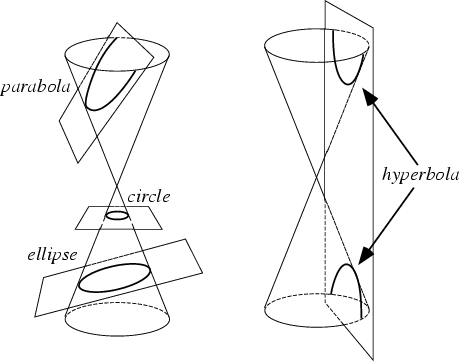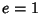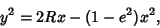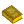## Conic SectionThe conic sections are the nondegenerate curves generated by the intersections of a Plane with one or two Nappes of a Cone. For a Plane parallel to a Cross-Section, a Circle is produced. The closed curve produced by the intersection of a single Nappe with an inclined Plane is an Ellipse or Parabola. The curve produced by a Plane intersecting both Nappes is a Hyperbola. The Ellipse and Hyperbola are known as Central Conics.

Because of this simple geometric interpretation, the conic sections were studied by the Greeks long before their application to inverse square law orbits was known. Apolloniuswrote the classic ancient work on the subject entitled On Conics. Keplerwas the first to notice that planetary orbits were Ellipses, and Newtonwas then able to derive the shape of orbits mathematically using Calculus, under the assumption that gravitational force goes as the inverse square of distance. Depending on the energy of the orbiting body, orbit shapes which are any of the four types of conic sections are possible.

A conic section may more formally be defined as the locus of a pointthat moves in the Plane of a fixed pointcalled the Focus and a fixed linecalled the Directrix (withnot on) such that the ratio of the distance offromto its distance fromis a constantcalled the Eccentricity. For a Focusand Directrix, the equation isIf, the conic is a Parabola, if, the conic is an Ellipse, and if, it is a Hyperbola.

In standard form, a conic section is writtenwhereis the Radius of Curvature andis the Eccentricity. Five points in a plane determine a conic (Le Lionnais 1983, p. 56).

See also Brianchon's Theorem, Central Conic, Circle, Cone, Eccentricity, Ellipse, Fermat Conic, Hyperbola, Nappe, Parabola, Pascal's Theorem, Quadratic Curve, Seydewitz's Theorem, Skew Conic, Steiner's Theorem

ReferencesConic Sections

Besant, W. H. Conic Sections, Treated Geometrically, 8th ed. rev. Cambridge, England: Deighton, Bell, 1890.

Casey, J. Special Relations of Conic Sections'' and Invariant Theory of Conics.'' Chs. 9 and 15 in A Treatise on the Analytical Geometry of the Point, Line, Circle, and Conic Sections, Containing an Account of Its Most Recent Extensions, with Numerous Examples, 2nd ed., rev. enl. Dublin: Hodges, Figgis, & Co., pp. 307-332 and 462-545, 1893.

Coolidge, J. L. A History of the Conic Sections and Quadric Surfaces. New York: Dover, 1968.

Coxeter, H. S. M. and Greitzer, S. L. Geometry Revisited. Washington, DC: Math. Assoc. Amer., pp. 138-141, 1967.

Downs, J. W. Conic Sections. Dale Seymour Pub., 1993.

Iyanaga, S. and Kawada, Y. (Eds.). Conic Sections.'' §80 in Encyclopedic Dictionary of Mathematics. Cambridge, MA: MIT Press, pp. 271-276, 1980.

Le Lionnais, F. Les nombres remarquables. Paris: Hermann, p. 56, 1983.

Lee, X. Conic Sections.'' http://www.best.com/~xah/SpecialPlaneCurves_dir/ConicSections_dir/conicSections.html

Ogilvy, C. S. The Conic Sections.'' Ch. 6 in Excursions in Geometry. New York: Dover, pp. 73-85, 1990.

Pappas, T. Conic Sections.'' The Joy of Mathematics. San Carlos, CA: Wide World Publ./Tetra, pp. 196-197, 1989.

Salmon, G. Conic Sections, 6th ed. New York: Chelsea, 1954.

Smith, C. Geometric Conics. London: MacMillan, 1894.

Sommerville, D. M. Y. Analytical Conics, 3rd ed. London: G. Bell and Sons, 1961.

Yates, R. C. Conics.'' A Handbook on Curves and Their Properties. Ann Arbor, MI: J. W. Edwards, pp. 36-56, 1952.